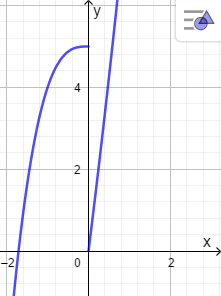# Consider the following function f(x) = \begin {cases} x^3+5, & \ \text{if} \ x \leq 0 \\ x^2...

## Question:

Consider the following function {eq}f(x) = \begin {cases} x^3+5, & \ \text{if} \ x \leq 0 \\ x^2 +8x, & \ \text{if} \ x>0 \end {cases} {/eq}

a. Find the critical numbers.

b. Find the open intervals on which function is increasing or decreasing.

## Critical Numbers

The Critical Numbers of a function can be values in which the function changes (it can end increasing and begin to decrease or finish decreasing and begin to increase). In the same sense, the intervals of increase or decrease of a function can be observed in a graph of the function.

We have the function

{eq}f(x) = \begin {cases} x^3+5, & \ \text{if} \ x \leq 0 \\ x^2 +8x, & \ \text{if} \ x>0 \end {cases} \\ {/eq}

Graph the functionWe can find the critical points by zeroing the first derivative or when the first derivative does not exist.

Differentiating the function {eq}f(x)=x^3+5\\ f'(x)=3x^{2} \\ {/eq}

{eq}f'(x)=0 {/eq} when {eq}x=0 {/eq}

Therefore,

a.Critical numbers at:

{eq}x=0 \\ {/eq}

b. Find the open intervals on which function is increasing or decreasing.

{eq}\begin{array}{r|D{.}{,}{5}} Interval & {-\infty<x<0} & {0<x<\infty} \\ \hline Test \space{} value & \ x=-1 & \ x=1 \\ Sign \space{} of \ f'(x) & \ f'(-1)>0 & \ f'(-1)>0 \\ Conclusion & increasing & increasing \\ \end{array} \\ \therefore \text{increasing's interval is } \space{ } \ (-\infty, \infty) {/eq}